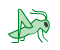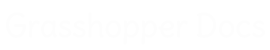﻿ Dodo - Addon for Grasshopper | Grasshopper DocsCommunity documentation for Grasshopper add-ons & plugins# Dodo

##### Dodo is a collection of tools for machine learning, optimization, and geometry manipulation. Regarding AI, it features neural networks, gradient descent, stochastic gradient descent and swarm optimization. Dodo has tools for scalar, vector and tensor fields manipulation which can be visualized using isosurfaces. Some components are implementations of papers, you can find more info and reference in their html description.
 Artificial Intelligence
 Field
 Graph
 kd-Tree
 MathNet
 Mesh
 Other
 Surface

### Field

 Construct Tensor Field (ConstructTF)Constructs a tensor field Custom Field (CustomField)Generate a user-defined vector field Deconstruct Tensor Field (DeconstructTensField)Deconstruct a tensor field into a connectivity mesh and a list of tensors Discrete Field (DiscrField)Generate a continuous field from a discrete vector set Field From Mesh (FieldFromMesh)Generates a field based on a mesh's principal curvature Field From Surface (FieldFromSurface)Generates a field based on a surface's principal curvature Field Potential (FieldPotential)Provided the field is conservative, this calculates the potential in each pointas the negative of the work done by a particle from the sampling point (A) to the source (B) Image Charge (ImageCharge)Create a field due to an image charge Point Trajectory (IntegFieldPoint)Find the trajectory of a point in a field Integrate Tensor Field (IntegrateTFMesh)Integrate Tensor Field on Mesh Path on Object (PathOnObj)Integrate a field using an object as a constraint Rotate Tensor Field (RotateTF)Description Smoothen Field (Smoothen a field)See html help in the component Iso-Potential Surface (IsoPotSurf)Calculates the iso-potential surfaces of a field Tensor Field - Vector (TFtoVF)Calculate a vector field from a tensor field and a single direction The resulting vector will be V(x) = TF(x) * D, where M is the tensor field in a point and D the direction given Tensor Field - Component (TFtoVF2)Creates a vector field by choosing a component of the Tensor Field. By default it takes the first direction. Right-click on the component to change that.

### kd-Tree

 Construct Node Value (ConKDNodeVal)Construct a kd-Node with a value associated to it Construct Node (ConKDNode)Construct a kd-Node Construct Tree (ConKDTree)Construct a kd-Tree Deconstruct Node (DeConKDNode)Deconstruct a kd-Node Nearest Neighbours Hypercube (NearKDNCube)Finds kdNodes in a hypercube Nearest Neighbours Distance (NearKDNNum)Finds kdNode within a certain distance from a given one Nearest Neighbours Number (NearKDNNum)Finds n nearest neighbours to the specified kdNode

### Mesh

 Convex Hull 3D (ConvexHull3D)Create a convex hull 3d from a poiont cloud Geodesic (GeodesicMesh)Geodesic on a mesh Integrate Mesh Curvature (IntegrateMshCrv)Integrates the principal directions field of a mesh Iso Mesh (IsoMesh)Creates and Iso Mesh Mesh Curvature (MeshCrv)Find the curvature field of a mesh Umbilic Points (UmbilicPoints)Find umbilc points on a mesh VoxelsSamples the field for creating the Voxels

### Other

 Dancing Banana (Banana)A funny dancing banana Series from List (SeriesList)Create a series of numbers having the same length of the given list Group Numbers (GroupNums)Group numbers Run Executable (RunExec)Run an executable with attributes Sort Curves (Sort curves)Sort curves along a given curve

### Graph

 Generate Graph (GenGraph)Create graph from curves Find Path (PathGraph)Visualize the shortest path Graph search (GraphSrch)Find distances in a graph Generate Network (GenGraph)Create graph from curves

### Artificial Intelligence

 Run Neural Network (RunNN)Run data through a trained neural network Supervised Training NN (SupTrainNN)Supervised training Unsupervised Training NN (UnsupTrainNN)Unsupervised training

### Surface

 Integrate Curvature FieldIntegrate Curvature Field

### MathNet

 Statistics (Stats)Calculate statistics for a series of numbers

## Video Tutorials

Site design © Robin Rodricks.   Dodo and associated data © 2022 Lorenzo Greco.
Rhinoceros and Grasshopper are registered trademarks of Robert McNeel & Associates.  Hosted by GitHub